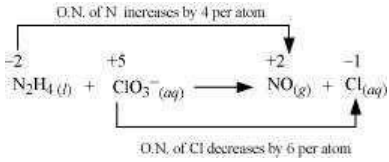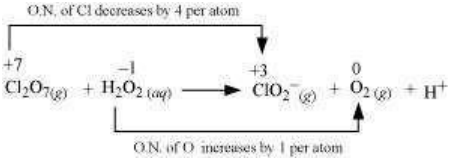8.19 Balance the following equations in basic medium by ion-electron method and oxidation number methods and identify the oxidising agent and the reducing agent.

(a) P4(s) + OH(aq) PH3(g) + HPO2 (aq)

(b) N2H4(l) + ClO3 (aq) NO(g) + Cl(g)

(c) Cl2O7 (g) + H2O2(aq) ClO2(aq) + O2(g) + H+

(a) The O.N. (oxidation number) of P decreases from 0 in  and increases from 0 in . Hence, ${\mathrm{P}}_{4}$ acts both as an oxidizing agent and a reducing agent in this reaction.
Ion-electron method:
The oxidation half equation is:
${\mathrm{P}}_{4\left(\mathrm{s}\right)}\to {\mathrm{HPO}}_{2\left(\mathrm{aq}\right)}^{-}$
The P atom is balanced as:
${\stackrel{0}{\mathrm{P}}}_{4\left(s\right)}\to 4\mathrm{H}\stackrel{2+}{\mathrm{P}}{\mathrm{O}}_{2}^{-}\left(\mathrm{aq}\right)$
The O.N. is balanced by adding 8 electrons as:
${\mathrm{P}}_{4\left(\mathrm{s}\right)}\to 4{\mathrm{HPO}}_{2\left(\mathrm{aq}\right)}^{-}+8{\mathrm{e}}^{-}$
The charge is balanced by adding $12{\mathrm{OH}}^{-}$ as:
${\mathrm{P}}_{4\left(\mathrm{s}\right)}+12{\mathrm{OH}}_{\left(\mathrm{aq}\right)}^{-}\to 4{\mathrm{HPO}}_{2\left(\mathrm{aq}\right)}^{-}+8{\mathrm{e}}^{-}$
The H and O atoms are balanced by adding $4{\mathrm{H}}_{2}\mathrm{O}$ as:
The reduction half equation is:
${\mathrm{P}}_{4\left(\mathrm{s}\right)}\to {\mathrm{PH}}_{3\left(\mathrm{g}\right)}$
The P atom is balanced as
$\stackrel{0}{{\mathrm{P}}_{4\left(\mathrm{s}\right)}}\to 4\stackrel{-3}{\mathrm{P}}{\mathrm{H}}_{3\left(\mathrm{g}\right)}$
The O.N. is balanced by adding 12 electrons as:
${\mathrm{P}}_{4\left(\mathrm{s}\right)}+12{\mathrm{e}}^{-}\to 4{\mathrm{PH}}_{3\left(\mathrm{g}\right)}$
The charge is balanced by adding $12{\mathrm{OH}}^{-}$ as:
${\mathrm{P}}_{4\left(\mathrm{s}\right)}+12{\mathrm{e}}^{-}\to 4{\mathrm{PH}}_{3\left(\mathrm{g}\right)}+12{{\mathrm{OH}}^{-}}_{\left(\mathrm{aq}\right)}$
The O and H atoms are balanced by adding $12{\mathrm{H}}_{2}\mathrm{O}$ as:
By muliplying equation (i) and 3 and (ii) with 2 and then adding them, the balanced chemical equation can be obtained as:
$5{\mathrm{P}}_{4\left(\mathrm{s}\right)}+12{\mathrm{H}}_{2}{\mathrm{O}}_{\left(\mathrm{l}\right)}+12{{\mathrm{HO}}^{-}}_{\left(\mathrm{aq}\right)}\to 8{\mathrm{PH}}_{3\left(\mathrm{g}\right)}+12{{\mathrm{HPO}}_{2}^{-}}_{\left(\mathrm{aq}\right)}$
(b)The oxidaion number of N increases from -2 in ${\mathrm{N}}_{2}{\mathrm{H}}_{4}$ to +2 in NO and the oxidation number of Cl decreases from +5 in ${\mathrm{ClO}}_{3}^{-}$ to -1 in ${\mathrm{Cl}}^{-}$. Hence, in this reaction, ${\mathrm{N}}_{2}{\mathrm{H}}_{4}$ is the reducing agent and ${\mathrm{ClO}}_{3}^{-}$ is the oxidizing agent.
Ion-electron method:
The oxidation half equation is:
${\stackrel{-2}{\mathrm{N}}}_{2}{H}_{4\left(l\right)}\to \stackrel{+2}{\mathrm{N}}{\mathrm{O}}_{\left(\mathrm{g}\right)}$
The N atoms are balanced as:
${\mathrm{N}}_{2}{\mathrm{H}}_{4\left(\mathrm{l}\right)}\to 2{\mathrm{NO}}_{\left(\mathrm{g}\right)}$
The oxidation number is balanced by adding 8 electrons as :
${\mathrm{N}}_{2}{\mathrm{H}}_{4\left(\mathrm{l}\right)}\to 2{\mathrm{NO}}_{\left(\mathrm{g}\right)}+8{\mathrm{e}}^{-}$
The charge is balanced by adding 8 ${\mathrm{OH}}^{-}$ ions as:
${\mathrm{N}}_{2}{\mathrm{H}}_{4\left(\mathrm{l}\right)}+8{{\mathrm{OH}}^{-}}_{\left(\mathrm{aq}\right)}\to 2{\mathrm{NO}}_{\left(\mathrm{g}\right)}+8{\mathrm{e}}^{-}$
The O atoms are balanced by adding $6{\mathrm{H}}_{2}\mathrm{O}$ as:
The reduction half equation is:
$\stackrel{+5}{\mathrm{C}}{{\mathrm{lO}}^{-}}_{3\left(\mathrm{aq}\right)}\to \stackrel{-1}{\mathrm{C}}{{\mathrm{l}}^{-}}_{\left(\mathrm{aq}\right)}$
The oxidation number is balanced by adding 6 electrons as:
${\mathrm{ClO}}_{3\left(\mathrm{aq}\right)}^{-}+6{\mathrm{e}}^{-}\to {\mathrm{Cl}}_{\left(\mathrm{aq}\right)}^{-}$
The charge is balanced by adding $6{\mathrm{OH}}^{-}$ ions as:
${\mathrm{ClO}}_{3\left(\mathrm{aq}\right)}^{-}+6{\mathrm{e}}^{-}\to {\mathrm{Cl}}_{\left(\mathrm{aq}\right)}^{-}+6{\mathrm{OH}}_{\left(\mathrm{aq}\right)}^{-}$
The O atoms are balanced by adding $3{\mathrm{H}}_{2}\mathrm{O}$ as:
The balanced equation can be obtained by multiplying equation (i) with 3 and equation (ii) with 4 and then adding them as:
$3{\mathrm{N}}_{2}{\mathrm{H}}_{4\left(\mathrm{l}\right)}+4{\mathrm{ClO}}_{3\left(\mathrm{aq}\right)}^{-}\to 6{\mathrm{NO}}_{\left(\mathrm{g}\right)}+4{\mathrm{Cl}}_{\left(\mathrm{aq}\right)}^{-}+6{\mathrm{H}}_{2}{\mathrm{O}}_{\left(\mathrm{l}\right)}$
Oxidation number method:
Total decrease in oxidation number of N = 2 x 4 = 8
Total increase in oxidation number of Cl = 1 x 6 = 6
On multiplying ${\mathrm{N}}_{2}{\mathrm{H}}_{4}$ with 3 and ${\mathrm{ClO}}_{3}^{-}$ with 4 to balance the increase and decrease in O.N., we get:
$3{\mathrm{N}}_{2}{\mathrm{H}}_{4\left(\mathrm{l}\right)}+4{\mathrm{ClO}}_{3\left(\mathrm{aq}\right)}^{-}\to {\mathrm{NO}}_{\left(\mathrm{g}\right)}+{\mathrm{Cl}}_{\left(\mathrm{aq}\right)}^{-}$
The N and  Cl atoms are balanced as:
$3{\mathrm{N}}_{2}{\mathrm{H}}_{4\left(\mathrm{l}\right)}+4{\mathrm{ClO}}_{3\left(\mathrm{aq}\right)}^{-}\to 6{\mathrm{NO}}_{\left(\mathrm{g}\right)}+4{\mathrm{Cl}}_{\left(\mathrm{aq}\right)}^{-}$
The O atoms are balanced by adding $6{\mathrm{H}}_{2}\mathrm{O}$ as:
$3 {\mathrm{N}}_{2}{\mathrm{H}}_{4\left(\mathrm{l}\right)}+4{\mathrm{ClO}}_{3\left(\mathrm{aq}\right)}^{-}\to 6{\mathrm{NO}}_{\left(\mathrm{g}\right)}+4{\mathrm{Cl}}_{\left(\mathrm{aq}\right)}^{-}+6{\mathrm{H}}_{2}{\mathrm{O}}_{\left(\mathrm{l}\right)}$
This is the required balanced equation.
(c)The oxidation number of Cl decreases from +7 in to +3 in ${\mathrm{ClO}}_{2}^{-}$ and the oxidation number of O increases from -1 in ${\mathrm{H}}_{2}{\mathrm{O}}_{2}$ to zero in ${\mathrm{O}}_{2}$. Hence, in this reaction, ${\mathrm{Cl}}_{2}{\mathrm{O}}_{7}$ is the oxidizing agent and ${\mathrm{H}}_{2}{\mathrm{O}}_{2}$ is the reducing agent.
Ion-electron method:
The oxidation half equation is:
${\mathrm{H}}_{2}{\stackrel{-1}{\mathrm{O}}}_{2\left(\mathrm{aq}\right)}\to {\stackrel{0}{\mathrm{O}}}_{2\left(\mathrm{g}\right)}$
The oxidation number is balanced by adding 2 electrons as:
${\mathrm{H}}_{2}{\mathrm{O}}_{2\left(\mathrm{aq}\right)}\to {\mathrm{O}}_{2\left(\mathrm{g}\right)}+2{\mathrm{e}}^{-}$
The charge is balanced by adding $2{\mathrm{OH}}^{-}$ ions as:
${\mathrm{H}}_{2}{\mathrm{O}}_{2\left(\mathrm{aq}\right)}+2{\mathrm{OH}}_{\left(\mathrm{aq}\right)}^{-}\to {\mathrm{O}}_{2\left(\mathrm{g}\right)}+2{\mathrm{e}}^{-}$
The oxygen atoms are balanced by adding $2{\mathrm{H}}_{2}\mathrm{O}$ as:
The reduction half equation is:
$\stackrel{+7}{\mathrm{C}}{l}_{2}{O}_{7\left(g\right)}\to \stackrel{+3}{\mathrm{C}}{\mathrm{lO}}_{2\left(\mathrm{aq}\right)}^{-}$
The Cl atoms are balanced as:
${\mathrm{Cl}}_{2}{\mathrm{O}}_{7\left(\mathrm{g}\right)}\to 2{\mathrm{ClO}}_{2\left(\mathrm{aq}\right)}^{-}$
The oxidation number is balanced by adding 8 electrons as:
${\mathrm{Cl}}_{2}{\mathrm{O}}_{7\left(\mathrm{g}\right)}+8{\mathrm{e}}^{-}\to 2{\mathrm{ClO}}_{2\left(\mathrm{aq}\right)}^{-}$
The charge is balanced by adding $6{\mathrm{OH}}^{-}$ as:
${\mathrm{Cl}}_{2}{\mathrm{O}}_{7\left(\mathrm{g}\right)}+8{\mathrm{e}}^{-}\to 2{\mathrm{ClO}}_{2\left(\mathrm{aq}\right)}^{-}+6{\mathrm{OH}}_{\left(\mathrm{aq}\right)}^{-}$
The oxygen atoms are balanced by adding $3{\mathrm{H}}_{2}\mathrm{O}$ as:
The balanced equation can be obtained by multiplying the equation (i) with 4 and adding equation (ii) to its as:
${\mathrm{Cl}}_{2}{\mathrm{O}}_{7\left(\mathrm{g}\right)}+6{\mathrm{H}}_{2}{\mathrm{O}}_{2\left(\mathrm{aq}\right)}+2{\mathrm{OH}}_{\left(\mathrm{aq}\right)}^{-}\to 2{\mathrm{ClO}}_{\left(\mathrm{aq}\right)}^{-}+4{\mathrm{O}}_{2\left(\mathrm{g}\right)}+5{\mathrm{H}}_{2}{\mathrm{O}}_{\left(\mathrm{l}\right)}$
Oxidation number method:
Total decrease in oxidation number of ${\mathrm{Cl}}_{2}{\mathrm{O}}_{7}=4×2=8$
Total increase in oxidation number of ${\mathrm{H}}_{2}{\mathrm{O}}_{2}=2×1=2$
By multiplying  with 4 to balance the increase and decrease in the oxidation number, we get:
${\mathrm{Cl}}_{2}{\mathrm{O}}_{7\left(\mathrm{g}\right)}+4{\mathrm{H}}_{2}{\mathrm{O}}_{2\left(\mathrm{aq}\right)}\to {\mathrm{ClO}}_{2\left(\mathrm{aq}\right)}^{-}+4{\mathrm{O}}_{2\left(\mathrm{g}\right)}$
The Cl atoms are balanced as:
${\mathrm{Cl}}_{2}{\mathrm{O}}_{7\left(\mathrm{g}\right)}+4{\mathrm{H}}_{2}{\mathrm{O}}_{2\left(\mathrm{aq}\right)}\to 2{\mathrm{ClO}}_{2\left(\mathrm{aq}\right)}^{-}+4{\mathrm{OH}}_{2\left(\mathrm{g}\right)}$
The O atoms are balanced by adding $3{\mathrm{H}}_{2}\mathrm{O}$as:
${\mathrm{Cl}}_{2}{\mathrm{O}}_{7\left(\mathrm{g}\right)}+4{\mathrm{H}}_{2}{\mathrm{O}}_{2\left(\mathrm{aq}\right)}\to 2{\mathrm{ClO}}_{2\left(\mathrm{aq}\right)}^{-}+4{\mathrm{O}}_{2\left(\mathrm{g}\right)}+3{\mathrm{H}}_{2}{\mathrm{O}}_{\left(\mathrm{l}\right)}$
The H atoms are balanced by adding  as:
${\mathrm{Cl}}_{2}{\mathrm{O}}_{7\left(\mathrm{g}\right)}+4{\mathrm{H}}_{2}{\mathrm{O}}_{2\left(\mathrm{aq}\right)}+2{\mathrm{OH}}_{\left(\mathrm{aq}\right)}^{-}\to 2{\mathrm{ClO}}_{2\left(\mathrm{aq}\right)}^{-}+4{\mathrm{O}}_{2\left(\mathrm{g}\right)}+5{\mathrm{H}}_{2}{\mathrm{O}}_{\left(\mathrm{l}\right)}$
This is the required balanced equation.